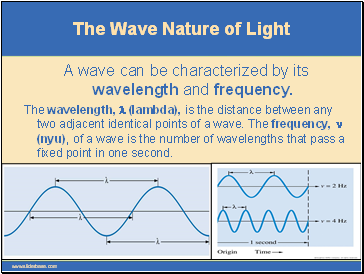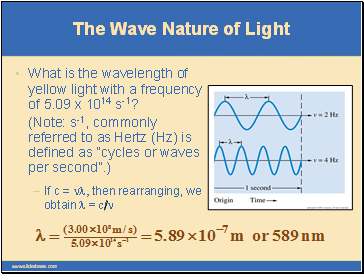# Quantum Theory of the AtomPage 1

#### WATCH ALL SLIDES

Slide 1Quantum Theory of the Atom

7.1 The Wave Nature of Light

7.2 Quantum Effects and Photons

7.3 The Bohr Theory of the Hydrogen Atom

Slide 2## The Wave Nature of Light

Presentation of Lecture Outlines, 7–2

A wave is a continuously repeating change or oscillation in matter or in a physical field. Light is also a wave.

It consists of oscillations in electric and magnetic fields that travel through space.Visible light, X rays, and radio waves are all forms of electromagnetic radiation.

Slide 3Presentation of Lecture Outlines, 7–3

The Wave Nature of Light

A wave can be characterized by its wavelength and frequency.

The wavelength, l (lambda), is the distance between any two adjacent identical points of a wave. The frequency, n (nyu), of a wave is the number of wavelengths that pass a fixed point in one second.

Slide 4Presentation of Lecture Outlines, 7–4

So, given the frequency of light, its wavelength can be calculated, or vice versa.

The Wave Nature of Light

The product of the frequency, n (waves/sec) and the wavelength, l (m/wave) would give the speed of the wave in m/s.

Slide 5Presentation of Lecture Outlines, 7–5

If c = nl, then rearranging, we obtain l = c/n

The Wave Nature of Light

What is the wavelength of yellow light with a frequency of 5.09 x 1014 s-1?

(Note: s-1, commonly referred to as Hertz (Hz) is defined as “cycles or waves per second”.)

Slide 6Presentation of Lecture Outlines, 7–6

Figure 7.5: The electromagnetic spectrum.

Slide 7Presentation of Lecture Outlines, 7–7

If c = nl, then rearranging, we obtain n = c/l.

What is the frequency of violet light with a wavelength of 408 nm?

The Wave Nature of Light

Slide 8